How Cheenta works to ensure student success?
Explore the Back-Story

# Differential Equation| IIT JAM 2014 | Problem 4Try this problem from IIT JAM 2014 exam. It requires knowledge of the exact differential equation and partial derivative.

## Differential Equation | IIT JAM 2014 | Problem 4

For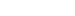, If the differential equation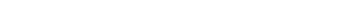is exact then

•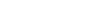•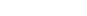•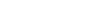•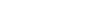### Key Concepts

Differential Equation

Exact D/E

Partial Differentiation

Answer:IIT JAM 2014 , Problem 4

Ordinary Differential Equations by Tenebaum and Pollard

## Try with Hints

Any first order and linear differential equation can be expressed as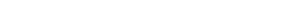where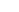and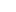are functions of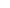and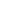.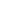is called exact if and only if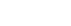Comparing the given equation withwe get,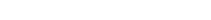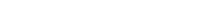Now can you calculate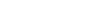?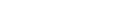[differentiating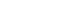with respect totakingas constant.]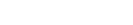[differentiating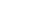with respect totakingas constant.]

Since the equation is exact ,theni.e.,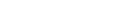Comparing the coefficients we get[ANS]

## Subscribe to Cheenta at Youtube

Try this problem from IIT JAM 2014 exam. It requires knowledge of the exact differential equation and partial derivative.

## Differential Equation | IIT JAM 2014 | Problem 4

For, If the differential equationis exact then

••••### Key Concepts

Differential Equation

Exact D/E

Partial Differentiation

Answer:IIT JAM 2014 , Problem 4

Ordinary Differential Equations by Tenebaum and Pollard

## Try with Hints

Any first order and linear differential equation can be expressed aswhereandare functions ofand.is called exact if and only ifComparing the given equation withwe get,Now can you calculate?[differentiatingwith respect totakingas constant.][differentiatingwith respect totakingas constant.]

Since the equation is exact ,theni.e.,Comparing the coefficients we get[ANS]

## Subscribe to Cheenta at Youtube

This site uses Akismet to reduce spam. Learn how your comment data is processed.

### Knowledge Partner